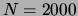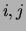Next: The SPLICE dataset: Feature Up: Classification with mixtures of Previous: The SPLICE dataset: Classification

The SPLICE dataset: Structure identification

Figure 17 presents a summary of the tree structures learned from thedataset in the form of a cumulated adjacency matrix. The adjacency matrices of the 20 graph structures obtained in the experiment have been summed. The size of the black square at coordinatesin the figure is proportional to the value of the-th element of the cumulated adjacency matrix. No square means that the respective element is 0. Since the adjacency matrix is symmetric, only half of the matrix is shown. From Figure 17 we see that the tree structure is very stable over the 20 trials. Variable 0 represents the class variable; the hypothetical splice junction is situated between variables 30 and 31. The figure shows that the splice junction (variable 0) depends only on DNA sites that are in its vicinity. The sites that are remote from the splice junction are dependent on their immediate neighbors. Moreover, examining the tree parameters, for the edges adjacent to the class variable, we observe that these variables build certain patterns when the splice junction is present, but are random and almost uniformly distributed in the absence of a splice junction. The patterns extracted from the learned trees are shown in Figure 18. The same figure displays the ``true'' encodings of the IE and EI junctions as given by watson:87. The match between the two encodings is almost perfect. Thus, we can conclude that for this domain, the tree model not only provides a good classifier but also discovers a model of the physical reality underlying the data. Note that the algorithm arrives at this result in the absence of prior knowledge: (1) it does not know which variable is the class variable, and (2) it does not know that the variables are in a sequence (i.e., the same result would be obtained if the indices of the variables were scrambled).Next: The SPLICE dataset: Feature Up: Classification with mixtures of Previous: The SPLICE dataset: Classification
Journal of Machine Learning Research 2000-10-19